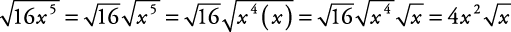## Simplifying Square Roots

##### Example 1

Simplify.

1. a, b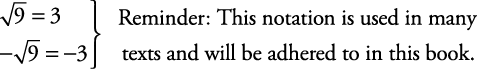2.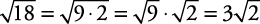3. If each variable is nonnegative (not a negative number),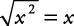If each variable could be positive or negative (deleting the restriction “If each variable is nonnegative”), then absolute value signs are placed around variables to odd powers.• e

If each variable is nonnegative,• f

If each variable is nonnegative,If each variable could be positive or negative, then you would write• g

If each variable is nonnegative,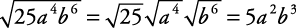If each variable could be positive or negative, you would write• h

If each variable is nonnegative,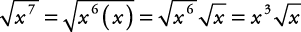If each variable could be positive or negative, you would write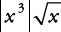• i

If each variable is nonnegative,• j

If each variable is nonnegative,